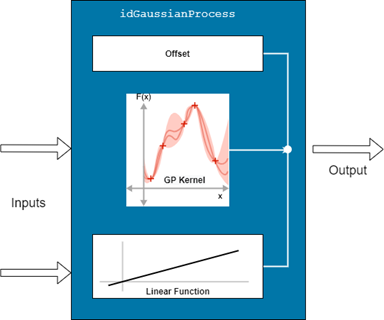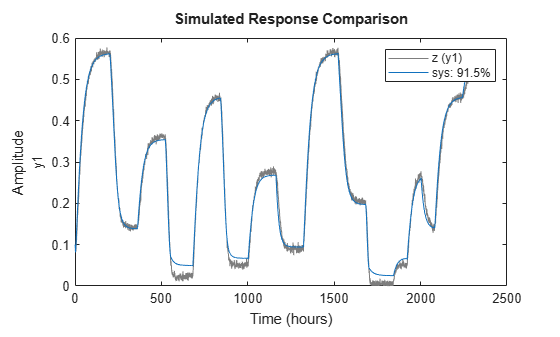# idGaussianProcess

Gaussian process regression mapping function for nonlinear ARX and Hammerstein-Wiener models (requires Statistics and Machine Learning Toolbox)

## Description

An `idGaussianProcess` object implements a Gaussian process (GP) regression model, and is a nonlinear mapping function for estimating nonlinear ARX and Hammerstein-Wiener models. This mapping object, which is also referred to as a nonlinearity, incorporates `RegressionGP` (Statistics and Machine Learning Toolbox) objects that the mapping function creates using Statistics and Machine Learning Toolbox™. The mapping object contains three components: an offset, a nonlinear component, which, in this case, is the GP kernel, and a linear component that uses a combination of linear weights.

The input to the mapping object can be an internal signal of a nonlinear black-box model, such as one of the following signals:

• Vector of the regressors of a nonlinear ARX model

• True model input of a Hammerstein-Wiener model

• Output of the linear block of a Hammerstein-Wiener modelMathematically, `idGaussianProcess` is a function that maps m inputs X(t) = [x(t1),x2(t),…,xm(t)]T to a scalar output y(t) using the following relationship:

`$y\left(t\right)={y}_{0}+Χ{\left(t\right)}^{T}PL+G\left(Χ\left(t\right),\theta \right)\right)$`

Here,

• X(t) is an m-by-1 vector of inputs, or regressors.

• y0 is the output offset, a scalar.

• P is an m-by-p projection matrix, where m is the number of regressors and p is the number of linear weights. m must be greater than or equal to p.

• L is a p-by-1 vector of weights.

• G(X,θ) is the regressive Gaussian process that constitutes the kernel of the `idGaussianProcess` object. G has a mean of zero and a covariance that the user specifies by choosing a kernel, and can be expressed generally as

`$G\left(X\right)=GP\left(0,K\left({X}_{test},{X}_{train},\theta \right)\right)$`

A zero-mean Gaussian process G predicts the output Ytest for a given input Xtest using the following relationship:

`$G\left({X}_{test}\right)=K\left({X}_{test},{X}_{train}\right){\left[K\left({X}_{train},{X}_{train}\right)+{\sigma }_{n}{}^{2}I\right]}^{-1}{Y}_{train}$`

Here:

• K(Xtest,Xtrain) is the covariance kernel function.

• Xtrain is a matrix representing the set of training inputs.

• Xtest is a matrix representing the set of test inputs.

• Ytrain is the vector of outputs from the training set.

• σn is the standard deviation of the additive measurement noise.

Gaussian process modeling is especially useful when you have only limited measurement data. For more information about creating GP regression models, see `fitrgp` (Statistics and Machine Learning Toolbox).

Use `idGaussianProcess` as the value of the `OutputFcn` property of an `idnlarx` model or the `OutputNonlinearity` property (but not the `InputNonlinearity` property) of an `idnlhw` object. For example, specify `idGaussianProcess` when you estimate an `idnlarx` model with the following command.

`sys = nlarx(data,regressors,idGaussianProcess)`
When `nlarx` estimates the model `sys`, it essentially estimates the parameters of the `idGaussianProcess` function.

You can use a similar approach when you specify input or output linearities using the `nlhw` command. For example, specify `idGaussianProcess` as an input nonlinearity with the following command.

`sys = nlhw(data,orders,idGaussianProcess,idSaturation)`

You can configure the `idGaussianProcess` function to disable components and fix parameters. To omit the linear component, set `LinearFcn.Use` to `false`. To omit the offset, set `Offset.Use` to `false`. To specify known values for the linear function and the offset, set their `Value` attributes directly and set the corresponding `Free` attributes to `False`. To modify the estimation options, set the option property in `EstimationOptions`. For example, to change the fit method to `'exact'`, use `G.EstimationOptions.FitMethod = 'exact'`. Use `evaluate` to compute the output of the function for a given vector of inputs.

## Creation

### Syntax

``G = idGaussianProcess``
``G = idGaussianProcess(kernelFunction)``
``G = idGaussianProcess(kernelFunction,kernelParameters)``
``G = idGaussianProcess(kernelFunction,kernelParameters,UseLinearFcn)``
``G = idGaussianProcess(kernelFunction,kernelParameters,UseLinearFcn,UseOffset)``

### Description

````G = idGaussianProcess` creates an `idGaussianProcess` object `G` with the kernel function `'SquaredExponential'` and default kernel parameters. The number of inputs is determined during model estimation and the number of outputs is 1. ```

example

````G = idGaussianProcess(kernelFunction)` specifies a specific kernel.```
````G = idGaussianProcess(kernelFunction,kernelParameters)` initializes the parameters of the specified kernel to the values in `kernelParameters`. ```

example

````G = idGaussianProcess(kernelFunction,kernelParameters,UseLinearFcn)` specifies whether the function uses a linear function as a subcomponent.```

example

````G = idGaussianProcess(kernelFunction,kernelParameters,UseLinearFcn,UseOffset)` specifies whether the function uses an offset term y0 parameter.```

### Input Arguments

expand all

Kernel covariance function, specified as character array or string. For information about the individual options, see Kernel (Covariance) Function in `fitrgp` (Statistics and Machine Learning Toolbox).

This argument sets the `G.Kernel.KernelFunction` property.

Initial values for the kernel parameters, specified as a vector. The size of the vector and the values depend on the choice of `kernelFunction`. For more information, see Kernel Parameters in `fitrgp` (Statistics and Machine Learning Toolbox).

This argument sets the `G.Kernel.Parameters.Value` property.

Option to use the linear function subcomponent, specified as `true` or `false`. This argument sets the value of the `G.LinearFcn.Use` property.

Option to use an offset term, specified as `true` or `false`. This argument sets the value of the `G.Offset.Use` property.

## Properties

expand all

Input signal names for the inputs to the mapping object, specified as a 1-by-m cell array, where m is the number of input signals. This property is determined during estimation.

Output signal name for the output of the mapping object, specified as a 1-by-1 cell array. This property is determined during estimation.

Properties of the GP kernel, specified as follows:

• `KernelFunction` —Covariance kernel function K, specified as one of the values listed in the `kernelFunction` argument description. For more information about these options, see Kernel (Covariance) Function in `fitrgp` (Statistics and Machine Learning Toolbox).

• `Parameters` — Parameters used in the kernel function, specified as the following properties:

• `Value` — Values of the kernel parameters, specified as a vector.

• `Names` — Names of the kernel parameters

• `InputProjection` — Projection matrix used to project inputs onto a lower dimensional subspace.

The size of the value vector and the values depend on the choice of `KernelFunction`. For more information, see Kernel Parameters in `fitrgp` (Statistics and Machine Learning Toolbox).

• `Free` — Option to estimate parameters, specified as a logical scalar. If all the parameters have finite values, such as when the `idGaussianProcess` object corresponds to a previously estimated model, then setting `Free` to `false` causes the parameters of the kernel G(X) to remain unchanged during estimation. The default value is `true`.

Parameters of the linear function, specified as follows:

• `Use` — Option to use the linear function in the `idGaussianProduct` model, specified as a scalar logical. The default value is `true`.

• `Value` — Linear weights that compose L', specified as a 1-by-p vector.

• `InputProjection` — Input projection matrix P, specified as an m-by-p matrix, that transforms the detrended input vector of length m into a vector of length p.

• `Free` — Option to update entries of `Value` during estimation, specified as a 1-by-p logical vector. The software honors the `Free` specification only if the starting value of `Value` is finite. The default value is `true`.

Parameters of the offset term, specified as follows:

• `Use` — Option to use the offset in the `idGaussianProcess` model, specified as a scalar logical. The default value is `true`.

• `Value` — Offset value, specified as a scalar.

• `Free` — Option to update `Value` during estimation, specified as a scalar logical. The software honors the `Free` specification of `false` only if the value of `Value` is finite. The default value is `true`.

Estimation options for the nonlinear block of the `idGaussianProcess` model, specified as follows. For more information on any of these options, see `fitrgp` (Statistics and Machine Learning Toolbox).

• `FitMethod` — Method to use for estimating the parameters of the `idGaussianProcess` nonlinear model, specified as one of the items in the following table.

Option Description
`'auto'`

Software selects the method automatically (default)

`'exact'`

Exact GP regression

`'sd'`

Subset of data points approximation

`'sr'`

Subset of regressors approximation

`'fic'`

Fully independent conditional approximation

• `ActiveSetMethod` — Active set selection method, specified as one of the items in the following table.

Option Description
`'random'`

Random selection (default)

`'sgma'`

Sparse greedy matrix approximation

`'entropy'`Differential entropy-based selection
`'likelihood'`

Subset of regressors log likelihood-based selection

• `SparseFitRegularization` — Regularization standard deviation for the sparse methods subset of regressors (`'sr'`) and the fully independent conditional approximation (`'fic'`), specified as a positive scalar value.

• `Optimizer` — Optimizer to use for parameter estimation, specified as one of the items in the following table.

Option Description
`'quasinewton'`Dense, symmetric rank-1-based, quasi-Newton approximation to the Hessian (default)
`'lbfgs'`LBFGS-based quasi-Newton approximation to the Hessian
`'fminsearch'`Unconstrained nonlinear optimization using the simplex search method of Lagarias et al. [see `fitrgp` (Statistics and Machine Learning Toolbox)]
`'fminunc'`Unconstrained nonlinear optimization (requires an Optimization Toolbox™ license)
`'fmincon'`Constrained nonlinear optimization (requires an Optimization Toolbox license)
• `OptimizerOptions` — Options for the optimizer, specified as a structure or object. When `Optimizer` is set or changed, the software automatically updates the value of `OptimizerOptions` to match the defaults for the corresponding optimizer. Use the properties of the `OptimizerOptions` option set to change the values from their defaults.

## Examples

collapse all

Load the input/output data from `twotankdata` and construct an `iddata` object `z`.

```load twotankdata u y z = iddata(y,u,0.8,'timeunit','hours');```

Create an `idGaussianProduct` mapping object `g` that uses a Matern kernel with the parameter 3/2.

`g = idGaussianProcess('Matern32');`

Estimate a nonlinear ARX model that uses `g` as the output function.

`sys = nlarx(z,[4 4 1],g)`
```sys = Nonlinear ARX model with 1 output and 1 input Inputs: u1 Outputs: y1 Regressors: Linear regressors in variables y1, u1 List of all regressors Output function: Gaussian process function using a Matern32 kernel Sample time: 0.8 hours Status: Estimated using NLARX on time domain data "z". Fit to estimation data: 97.14% (prediction focus) FPE: 2.82e-05, MSE: 2.795e-05 ```

Display the postestimation properties of `g`.

`disp(sys.OutputFcn.Input)`
```Function inputs Name: {1x8 cell} Mean: [-4.7062e-17 -5.3807e-17 -5.2324e-17 -8.3748e-18 1.0174e-15 ... ] Range: [2x8 double] ```
`disp(sys.outputFcn.Offset)`
```Output Offset: initialized to -8.11e-17 Use: 1 Value: -8.1058e-17 Free: 1 ```
`disp(sys.outputFcn.NonlinearFcn)`
```GP kernel and its parameters KernelFunction: 'Matern32' Parameters: '<Kernel parameters>' Free: 1 Inputs: {1x8 cell} Outputs: {'y1(t):Nonlinear'} ```

Compare the output of `sys` with the measured output `z`.

`compare(z,sys)`The nonlinear model shows a good fit to the estimation data.

Load the data `z3`.

`load iddata3 z3`

Create an `idGaussianProcess` object `G` that sets the `UseLinearFcn` argument to `0`. Since this argument is third in the syntax, you must also specify `kernelFunction` and `kernelParameters`. Set `kernelFunction` to its default value of `'SquaredExponential'`. Set the `kernelParameters` argument to `[]`, which specifies no initialization for the parameters.

```kernelFunction = 'SquaredExponential'; kernelParameters = []; UseLinearFcn = 0; G = idGaussianProcess(kernelFunction,kernelParameters,UseLinearFcn)```
```G = Gaussian Process Function Nonlinear Function: Gaussian process function using a SquaredExponential kernel Linear Function: not in use Output Offset: uninitialized Inputs: {1x0 cell} Outputs: {1x0 cell} Kernel: 'GP kernel and its parameters' LinearFcn: 'Linear function parameters' Offset: 'Offset parameters' EstimationOptions: 'Estimation option set' ```

The properties of `G` are consistent with your inputs.

Load the data `z3`.

`load iddata3 z3`

Create an `idGaussianProcess` object that has no offset block by first creating a default object, and then, using dot notation to set the `G.Offset.Use` property directly.

```G = idGaussianProcess; G.Offset.Use = 0```
```G = Gaussian Process Function Nonlinear Function: Gaussian process function using a SquaredExponential kernel Linear Function: uninitialized Output Offset: not in use Inputs: {1x0 cell} Outputs: {1x0 cell} Kernel: 'GP kernel and its parameters' LinearFcn: 'Linear function parameters' Offset: 'Offset parameters' EstimationOptions: 'Estimation option set' ```

The function description identifies the output offset as `not in use`.

## Version History

Introduced in R2021b

expand all

Not recommended starting in R2022a

Behavior changed in R2022a